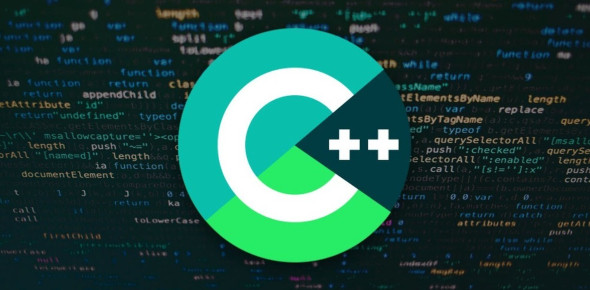# C++ Practice Test Quiz!

22 Questions | Total Attempts: 516Settings.

• 1.
The basic commands that a computer performs are ____, and performance of arithmetic and logical operations.
• A.

Input, file, list

• B.

Input, output, storage

• C.

Output, folder, storage

• D.

Storage, directory, log

• 2.
A program called a(n) ____ translates instructions written in high-level languages into machine code.
• A.

Assembler

• B.

Decoder

• C.

Compiler

• D.

• 3.
Suppose that x is an int variable. Which of the following expressions always evaluates to true?
• A.

(x > 0) || ( x

• B.

(x > 0) && ( x

• C.

(x >= 0) || (x == 0)

• D.

(x > 0) && (x == 0)

• 4.
Which of the following expressions correctly determines that x is greater than 10 and less than 20?
• A.

10 < x < 20

• B.

(10 < x < 20)

• C.

10 < x || x < 20

• D.

10 < x && x < 20

• 5.
What is the output of the following C++ code? int x = 35; int y = 45; int z; if (x > y) z = x + y; else z = y – x; cout << x << " " << y << " " << z << endl;
• A.

35 45 80

• B.

35 45 –10

• C.

35 45 10

• D.

35 45 0

• 6.
Suppose that x, y, z, and w are int variables, and x = 3, y = 4, z = 7, and w = 1; What is the output of the following statement?  cout << " x == y : " << (x == y ) << endl;
• A.

X == y: 0

• B.

X != z : 1

• C.

Y == z – 3: 1

• D.

! (z > w): 0

• 7.
Suppose that x, y, z, and w are int variables, and x = 3, y = 4, z = 7, and w = 1; What is the output of the following statement?  cout << " ! (z > w ) : " << ! (z > w ) << endl;
• A.

X != z : 1

• B.

X == y: 0

• C.

Y == z – 3: 1

• D.

! (z > w): 0

• 8.
The type of error in the following statement is : int a=b/0     //  b is defined as int
• A.

Syntax error

• B.

Logoical error

• C.

Run-time error

• D.

Compilation error

• 9.
The driver function of a C++ program that if it is not present in a program, no execution can take place:
• A.

Main

• B.

#include directive

• C.

Using namespace

• D.

Std

• 10.
What is the output of the following code : int main() { int a=4 ; (a>0)? cout<< " Number is positive " : cout<< " Number is negative " ; return 0 ; }
• A.

True

• B.

False

• C.

Number is positve

• D.

Number is negative

• 11.
What is the output of the following code : int main() { int num = 9; if (num<0); num= -num ; return o ; }
• A.

9

• B.

-9

• C.

0

• D.

Syntax error

• 12.
The output of the following code is : int main() { int n=17 ; cout << ( n == 16 ) << endl ; return 0 ; }
• A.

N=16

• B.

16

• C.

(n=16)

• D.

(n==16)

• 13.
A C++ code line ends with?
• A.

A Semicolon (;)

• B.

A full stop (.)

• C.

A comma ( ,)

• D.

Slash (/)

• 14.
What is the output of the following code: int main() { int x=7 ; if ( x=8)   cout << x-1 ; else  cout << x+1 ; return 0 ; }
• A.

8

• B.

6

• C.

7

• D.

Syntax error

• 15.
What is the output of the following code: int main () { int x=2 , y ; //x=x*2 ; y=x++ ; cout << "x= " << x << "\ty="  << y ; return 0 ; }
• A.

X = 4 y = 5

• B.

X = 3 y = 2

• C.

X = 2 y= 3

• D.

X = 5 y = 4

• 16.
What is the output of the following code: int main() { int a=98 ; double b=5 ; cout << a/3.0 ; return 0 ; }
• A.

32.6667

• B.

32

• C.

33

• D.

32.66

• 17.
Which of the following is a unary operator?
• A.

*

• B.

++

• C.

%

• D.

/

• 18.
Which of the following is not a relational operator in the C++ program?
• A.

=

• B.

==

• C.

>=

• D.

• 19.
C++ program may have more than one main function?
• A.

True

• B.

Fualse

• 20.
Which of the following is not a standard data tybe ?
• A.

Int

• B.

Char

• C.

Float

• D.

Data

• 21.
The output of the following code is : int main() { float x=1 ; cout << ( x == 3.0 / 7.0 + 2.0 / 7.0 + 2.0 / 7.0 ) ; return 0 ; }
• A.

1.0

• B.

0.999999

• C.

True

• D.

None of above

• 22.
Which of the following is illegal identifier ?
• A.

EmoName

• B.

X2

• C.

X_Y

• D.

Return

Related TopicsBack to top